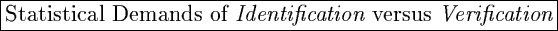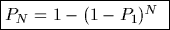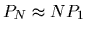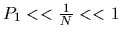John Daugman, University of Cambridge

It is useful to examine the relationship between False Accept probabilities when attempting a verification (a one-to-one comparison against a single stored template), versus an identification (a one-to-many comparison against all the enrolled biometric templates in some database of size N). In this analysis, we assume that the presenting template is not one of those enrolled, and so we only consider False Accept probabilities. (Extension to the case of an enrolled template is straightforward.)

Let P1 = probability of a False Accept in verification trials.

Let PN = probability of a False Accept in identification trials after exhaustive search through a database of size N. We wish to compute this.

Clearly the probability of not getting a False Accept on any given trial is (1-P1). This must happen N independent times, and so the probability of it not occuring on any of those N trials is (1-P1)N. Thus the probability of making at least one False Accept among those N trials is just one minus that probability:Example: Consider a biometric verifier that achieves a 99.9% Correct Rejection performance in verifications. Thus P1 = 0.001 (one False Accept in 1,000 trials). How will it perform when searching through a database?

Using the above expression, we see that for the following database sizes N, these will be the probabilities PN that this verifier makes at least one False Accept:
• Database of 200: PN = 0.181 (False Accept probability 18%)
• Database of 2,000: PN = 0.86 (False Accept probability 86%)
• Database of 10,000: PN = 0.99995 (False Accept probability 99.995%)
Note that once the enrolled database size reaches about 7,000 persons, this verifier becomes more likely (99.91%) to produce a False Accept in identification trials than it is to produce a Correct Rejection (99.9%) in verification trials!

Conclusion: Identification is vastly more demanding than verification, and even for moderate database sizes, merely good" verifiers are of no use as identifiers. Observing the approximation thatfor small, when searching a database of size N an identifier needs to be roughly N times better than a verifier to achieve comparable odds against a False Accept.